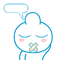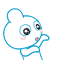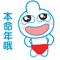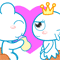# python 正无穷（float(" inf")）和负无穷（float("-inf")）的简介【基础知识】

【摘要】在 python 中可以用 float("+inf") 或者 float("inf") 来表示正无穷，也可以用 float("-inf") 来表示负无穷，本文就 python 的正无穷和负无穷进行相关的讲解。

1. `>>> a = float('+inf')`
2. `>>> a,type(a)`
3. `(inf, <class 'float'>)`
4. `>>> b = float('+inf')`
5. `>>> b,type(b)`
6. `(inf, <class 'float'>)`
7. `# 正无穷 = 正无穷（负无穷同理）`
8. `>>> a == b`
9. `True`
10. `# 正无穷 + 正无穷 = 正无穷（负无穷同理）`
11. `>>> c = a + b`
12. `>>> a == c`
13. `True`
14. `# 正无穷 + 任意正数 = 正无穷（负无穷同理）`
15. `>>> d = 1 + float('+inf')`
16. `>>> a == d`
17. `True`
18. `# 正无穷 + 任意负数 = 正无穷（负无穷同理）`
19. `>>> e = -999999999999 + float('+inf') `
20. `>>> a == e`
21. `True`
22. `# 正无穷大于所有数（负无穷小于所有数）`
23. `>>> float('+inf') > 99999999999999999999999`
24. `True`
25. `>>> float('-inf') < -99999999999999999999999`
26. `True`
27. `# 正无穷 * 任意正数 = 正无穷`
28. `>>> float('inf') * 99999999999`
29. `inf`
30. `# 正无穷 * 任意负数 = 负无穷`
31. `>>> float('inf') * -99999999999 `
32. `-inf`
33. `# 正无穷、负无穷 * 0 会得到 not a number（nan）`
34. `>>> float('inf') * 0           `
35. `nan`
36. `>>> float('-inf') * 0 `
37. `nan`
38. `# 正无穷 / 正无穷得到 not a number（nan）`
39. `>>> float('+inf') / float('+inf')            `
40. `nan`
41. `# 所有正数 / 正无穷 = 0.0`
42. `>>> 999999999999999999999999 / float('+inf') `
43. `0.0`
44. `# 0 / 正无穷 = 0.0`
45. `>>> 0 / float('+inf')                         `
46. `0.0`
47. `# 所有负数 / 正无穷 = -0.0`
48. `>>> -999999999999999999999999 / float('+inf') `
49. `-0.0`
50. `# 0 / 负无穷 = -0.0`
51. `>>> 0 / float('-inf') `
52. `-0.0`

0

0

 评论审核未开启 回复后邮件通知我• 浏览最多
• 评论最多